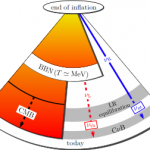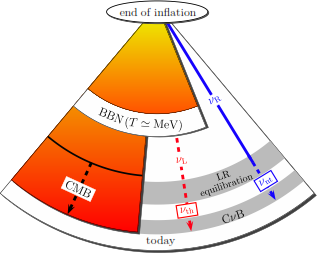# Paper of the month:  Non-thermal cosmic neutrino backgroundThe Cosmic Neutrino Background (CNB) is a solid prediction of the Standard Models of Particle Physics and Cosmology. In the early universe, neutrinos were formed as part of the thermal bath, a hot plasma filling the universe (thermal production). As the universe expanded and cooled down to MeV temperatures, the neutrinos decoupled from the thermal bath, traveling freely through space ever since. Neutrinos are very weakly interacting particles, which makes the detection of this neutrino background very challenging and so far experimentally inaccessible. However, on the other hand, this same property implies that once we succeed in measuring the cosmic neutrino background, we can not only learn something about the properties of neutrinos but also the CNB is a window to the very early universe, back to the times of the formation of light elements in Big Bang Nucleosynthesis (BBN), when the neutrinos decoupled from the thermal bath at about two minutes after the “Big Bang”. For comparison, the cousin of the CNB, the better-known Cosmic Microwave Background (CMB), consisting of background photons instead of neutrinos, had lead to major breakthroughs in modern cosmology. This window however only leads back to temperature around 1 eV, nearly 400,000 years later than the CNB window. This paper discusses how physics beyond the standard model, in particular non-thermally generated right-handed neutrinos, can modify the CNB predictions.

#### October 15, 2015 by tiinatimonen

Neutrinos have the unique property that they can be either Majorana or Dirac particles. Since neutrinos are massive particles, if neutrinos are Dirac they should pair up with light right-handed neutrinos, which are complete singlets under the Standard Model gauge group, i.e. as long as they are ultra-relativistic (only then left- and right-handed particles are distinguishable), they do not interact with any other particle. Once they become non-relativistic, the left -and right-handed neutrino population will be equalized. In the standard picture of the CNB, only left-handed neutrinos are produced in the thermal bath. For the Dirac neutrino scenario, which is the scenario we will focus on in the following, as the universe expands and the neutrino temperature cools down, half of these are converted into invisible right-handed neutrinos, thus halving the expected counting rate in any CNB detection experiment. In the Majorana case, there is only one type of light neutrino (there could be additional species of heavy right-handed neutrinos, but these would decay before the present time) and hence the counting rate in a CNB experiment would be twice that of the Dirac case.

In this paper, the authors discuss the possibility that there is an additional source for neutrinos in the early universe, namely that right-handed neutrinos are produced non-thermally, for example in the decay of the inflaton particle. These neutrinos never thermalize, but travel freely through the universe. At late times, half of this population is transformed into (detectable) left-handed particles, yielding an irreducible background in any CNB detection experiment.

There are constraints on this scenario. In particular, the size of this additional contribution to the energy density of the universe is bounded by observational constraints on BBN and the CMB. Nevertheless, the contribution can be sizable: The number density of non-thermally sourced neutrinos today can be as large as 217 neutrinos per cubic centimeter, which is 65 % of the number density predicted for the CNB and 53% of the photon density of the CMB.1

This has several interesting consequences. Firstly, this is a potential irreducible background which any experiment aiming at detecting the CNB (e.g. the proposed PTOLEMY experiment) will have to take into account. While increasing the overall detection probability, it will be challenging to disentangle the two components and hence the early universe physics associated with them. Moreover, taking this effect into account diminishes the difference between Dirac and Majorana neutrinos in the CNB: For Dirac neutrinos the rate was considered to be half as large as for Majorana neutrinos (since half of the energy density is converted into invisible right-handed neutrinos), yielding a possibility to distinguish the two. If there is a sizable non-thermal source, this difference is smaller (though still present). On the other side, a non-thermal source would also imply an exciting window to the even earlier early universe, for instance when the universe was supposedly inflating. Since these neutrinos never thermalize, they still carry information about their source, which will lie at times much before the decoupling of the left-handed neutrinos from the thermal bath. This is illustrated in Fig. 1.Fig 1: Windows to the early universe: CMB, CNB and non-thermal neutrino background. Taken from arxiv:1509.00481 [hep-ph].

1 It should be noted that there are two important assumptions leading to these numbers: (i) the absence of a Majorana mass term for the neutrinos and (ii) the non-thermally sourced neutrinos form a degenerate Fermi gas today.

Text by Valerie Domcke and Pedro Machado